﻿ 关于合成孔径雷达图像的量化位数和量化方法选择

# 关于合成孔径雷达图像的量化位数和量化方法选择Choosing the Quantization Bit and Quantization Method of Synthetic Aperture Radar Image

Abstract: After the echoes of micro SAR (Synthetic Aperture Radar) equipment are focused, in order to facilitate storage and secondary processing, an effective down-sampling method is studied to increase the image processing rate while reducing the loss of image quality. This article briefly introduces the selection of micro-miniaturized synthetic aperture radar image storage bits, analyzes the relationship between image storage bits and image quality from two aspects of read rate and algorithm processing efficiency, and elaborates the synthetic aperture radar image down sampling method in detail, and simulates the signal-to-noise ratio and quantization noise corresponding to different quantization methods. According to the simulation results, the non-uniform quantization method proposed in the article is a down sampling method that can improve the volume-to-noise ratio.

1. 引言

2. 轻小型化SAR存储位数的选择

2.1. SAR系统结构对存储位数选择有影响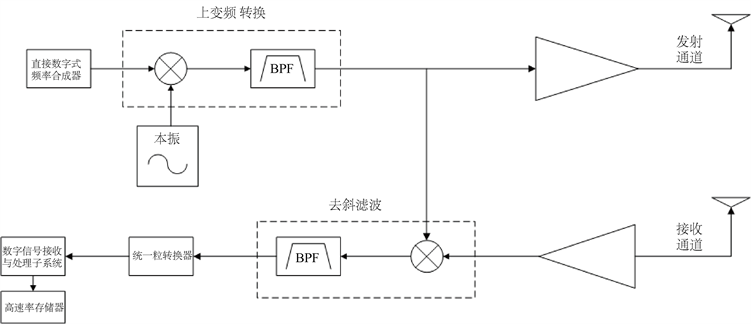Figure 1. Schematic diagram of continuous wave radar structure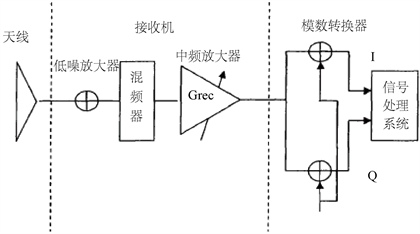Figure 2. Diagram of the various parts of the synthetic aperture radar system

2.2. 量化位数的改变对信噪比带来的影响

2.2.1. 量化信噪比

${V}_{\text{A/D}}=\frac{{V}_{\text{LSB}}}{\sqrt{12}}$

${N}_{q}=E\left[{\left({m}_{k}-{m}_{q}\right)}^{2}\right]=\underset{a}{\overset{b}{\int }}{\left({m}_{k}-{m}_{q}\right)}^{2}f\left({m}_{k}\right)\text{d}{m}_{k}=\underset{i=1}{\overset{M}{\sum }}\underset{{m}_{i-1}}{\overset{{m}_{i}}{\int }}{\left({m}_{k}-{q}_{i}\right)}^{2}f\left({m}_{k}\right)\text{d}{m}_{k}$

$SNR=\frac{{S}_{0}}{{N}_{q}}=\frac{E\left({m}_{k}^{2}\right)}{E\left[{\left({m}_{k}-{m}_{q}\right)}^{2}\right]}=\frac{\underset{a}{\overset{b}{\int }}{m}_{k}^{2}f\left({m}_{k}\right)\text{d}{m}_{k}}{\underset{a}{\overset{b}{\int }}{\left({m}_{k}-{m}_{q}\right)}^{2}f\left({m}_{k}\right)\text{d}{m}_{k}}=\frac{\underset{a}{\overset{b}{\int }}{m}_{k}^{2}f\left({m}_{k}\right)\text{d}{m}_{k}}{\underset{i=1}{\overset{M}{\sum }}\underset{{m}_{i-1}}{\overset{{m}_{i}}{\int }}{\left({m}_{k}-{q}_{i}\right)}^{2}f\left({m}_{k}\right)\text{d}{m}_{k}}$

2.2.2. 目标检测算法速度Table 1. Detection speed result data

3. 存储位数变换方法

3.1. 基于均匀量化位数变换方法

$\begin{array}{l}{R}_{1}=\left(-\infty ,a\right]\\ {R}_{2}=\left(a,a+\Delta \right]\\ {R}_{3}=\left(a+\Delta ,a+2\Delta \right]\\ \text{\hspace{0.17em}}\text{\hspace{0.17em}}\text{\hspace{0.17em}}\text{\hspace{0.17em}}\text{\hspace{0.17em}}⋮\\ {R}_{N}=\left(a+\left(N-2\right)\Delta ,+\infty \right]\end{array}$

$\Delta v=\frac{b-a}{M}$

${m}_{i}=a+i\Delta v,\text{\hspace{0.17em}}\text{\hspace{0.17em}}i=0,1,\cdots ,M$

${q}_{i}=\frac{{m}_{i}+{m}_{i-1}}{2},\text{\hspace{0.17em}}\text{\hspace{0.17em}}i=1,2,\cdots ,M$

64位转换为8位，基于通用计算机软件方法。

16位转换为8位，专用SAR成像处理系统。

${v}_{qn}=\sqrt{\frac{1}{Q}\underset{-\frac{Q}{2}}{\overset{+\frac{Q}{2}}{\int }}{x}^{2}\text{d}x}=\sqrt{\frac{1}{Q}{\left[\frac{{x}^{3}}{3}\right]|}_{-\frac{Q}{2}}^{+\frac{Q}{2}}}=\sqrt{\frac{{Q}^{2}}{{2}^{3}×3}+\frac{{Q}^{2}}{{2}^{3}×3}}=\frac{Q}{\sqrt{12}}$

3.2. 基于非均匀压缩的位数变换方法

A律对数量化器：

$f\left(x\right)=\left\{\begin{array}{l}\frac{Ax}{1+\mathrm{ln}A},\text{\hspace{0.17em}}\text{\hspace{0.17em}}\text{ }\text{\hspace{0.17em}}0\le x\le 1/A\\ \frac{1+\mathrm{ln}Ax}{1+\mathrm{ln}A},\text{\hspace{0.17em}}\text{\hspace{0.17em}}1/A\le x\le 1\end{array}$

4. 基于MATLAB的仿真实验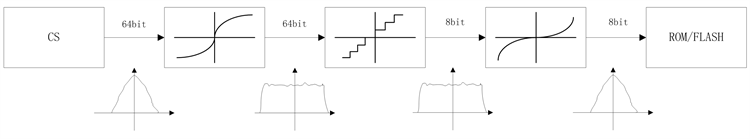Figure 3. Schematic diagram of non-uniform compressed bit transformation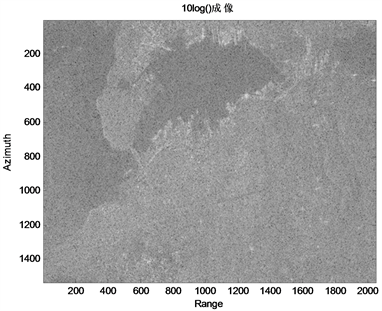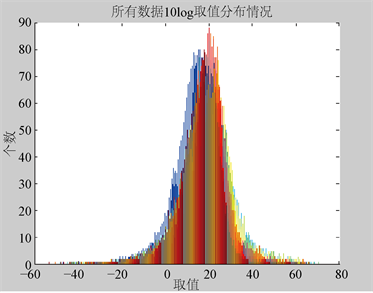Figure 5. All image data distribution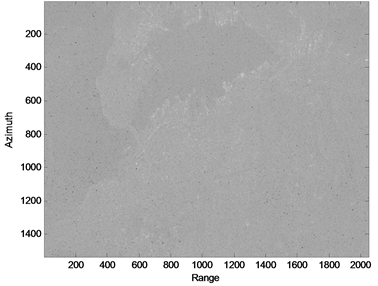Figure 6. 8 bit uniform quantization result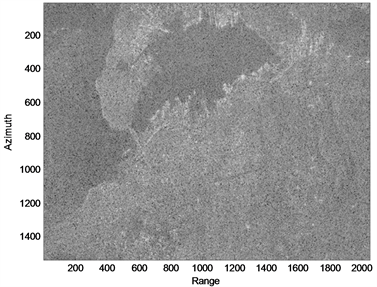Figure 7. 8-bit non-uniform quantization result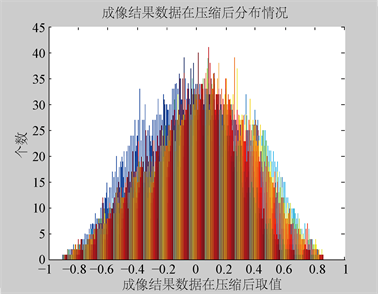Figure 8. Compression of all image dataTable 2. Comparison of different quantitative results

5. 结束语

 Lan. G. Cumming, Frank. H. Wong. 合成孔径雷达成像: 算法与实现[M]. 北京: 电子工业出版社, 2012.

 韩晓东, 贾小雪, 冯锦, 等. 基于水云模型的SAR图像量化增强算法[J]. 科学技术与工程, 2013, 13(19): 5549-5554.

 姜明. 机载SAR图像量化及去噪声算法的工程应用研究[J]. 空载雷达, 2008(2): 17-20, 11.

 陈海波. SAR量化饱和分析与校正技术研究[D]: [硕士学位论文]. 北京: 中国科学院研究生院(电子学研究所).

 Jin, M., Cheng, T. and Chen, M. (2002) Chirp Scaling Algorithms for SAR Processing. Proceedings of IGARSS’93 - IEEE International Geoscience and Remote Sensing Symposium, Tokyo, 18-21 August 1993, 1169-1172.

 王宇, 张志敏, 邓云凯. SAR实时方位预处理中的量化误差分析[J]. 现代雷达, 2006(2): 36-39.

 吴红军, 皮亦鸣. 微型SAR成像量化显示的FPGA实现[J]. 电讯技术, 2009, 49(11): 27-30.

Top